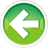# 8.2 — Value categories (r-values and l-values)

Before we talk about our first compound type (l-value references), we’re going to take a little detour and talk about what an `l-value` is.

In C++, every expression has two properties: a `type` and a `value category`. Let’s explore these concepts in more detail.

The type of an expression

In lesson 1.9 -- Introduction to expressions, we defined an expression as, “a combination of literals, variables, operators, and explicit function calls (not shown above) that produce a single output value”. This “output value” is actually an object that contains both a value and a type.

The type of an expression is equal to the type of the value that results from the resolved expression.

You’ve already seen examples of the `type` property of expressions:

Variables `v1` and `v2` have initializers that are simple expressions.

For `v1`, the compiler will determine (at compile time) that a division with two `int` operands will produce an `int` result, so `int` is the type of this expression. Via type inference, that `int` type will then be used as the type of `v1`.

For `v2`, the compiler will determine (at compile time) that a division with a `double` and an `int` operand will produce a `double` result. Remember that arithmetic operators must have operands of matching types, so in this case, the `int` operand gets promoted to a `double`, and a floating point division is performed. So `double` is the type of this expression.

Compound expressions (those with multiple operators) work the same way -- the compiler uses the precedence and associativity rules to determine the order in which the expression will evaluate. The type of the resulting value is the type of the expression.

Note that the type of an expression can always be determined at compile time (otherwise type deduction wouldn’t work) -- however, the value of the expression may be determined at either compile time (if the expression is constexpr) or runtime (if the expression is not constexpr).

Value categories

Now consider the following program:

``` int main() { int x{};```

``` x = 5; // valid 5 = x; // error ```

``` return 0; } ```

One of these assignment statements is valid (assigning value `5` to variable `x`) and one is not (what would it mean to assign the value of `x` to the literal value `5`?). So how does the compiler know which expressions can legally appear on either side of an assignment statement?

The answer lies in the second property of expressions: the `value category`. An expression’s value category helps the compiler determine how to create, copy, and move objects during the evaluation of the expression.

Since assignments are a type of copy operation, the value category is used to determine whether a given assignment is legal.

Prior to C++11, C++ had only two value categories. In this lesson, we’ll explore this simplified (pre-C++11) view of two most common ones (`l-values` and `r-values`), which is all we need for now.

As an aside...

In C++11, three more value categories were added (to bring the total to five). These additional value categories are used to facilitate `move semantics`, which is a topic we’ll cover in a future chapter.

L-values

An l-value (short for “locator value”, or “left value”, and sometimes written as `lvalue`) is an expression that results in an object with an identifiable memory address. Variables are an example of an l-value, as every variable has a discrete memory address.

The expression on the left-hand side of an assignment must be an l-value expression:

Since the introduction of constants into the language, l-values now come in two subtypes: a modifiable l-value is an l-value whose value can be modified. A non-modifiable l-value is an l-value whose value can’t be modified (because the l-value is const or constexpr).

Non-modifiable l-values are not legal targets for being assigned to, so it’s more accurate to say that the left-hand side of an assignment must be a modifiable l-value.

We can now answer the question of why `5 = x;` is not valid -- because the left-hand side of an assignment must be a modifiable l-value, and `5` is not an l-value at all.

So what is `5`?

R-values

The opposite of l-values are `r-values` (pronounced arr-values). R-values are defined by negation: an r-value is any expression that is not an l-value. Commonly seen r-values include most literals (string literals are an exception) and functions returning values by copy, neither of which have an identifiable memory address (they are temporary values).

In an expression, r-values are evaluated for their values:

Functions that return values by copy are also considered r-values:

L-value to r-value conversion

L-values will implicitly convert to r-values (and be evaluated for their values), which is why an expression like this works fine:

Now consider this snippet:

In this statement, the variable `x` is being used in two different contexts. On the left side of the assignment operator, `x` is being used as an l-value (variable with an address). On the right side of the assignment operator, `x` is being used as an r-value, and will be evaluated to produce a value. When C++ evaluates the above statement, it evaluates as:

Which makes it obvious that C++ will assign the value `3` back into variable `x`.

Now that we’ve covered l-values, we can get to our first compound type: the `l-value reference`.8.3 -- L-value referencesIndex8.1 -- Introduction to compound data types